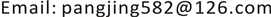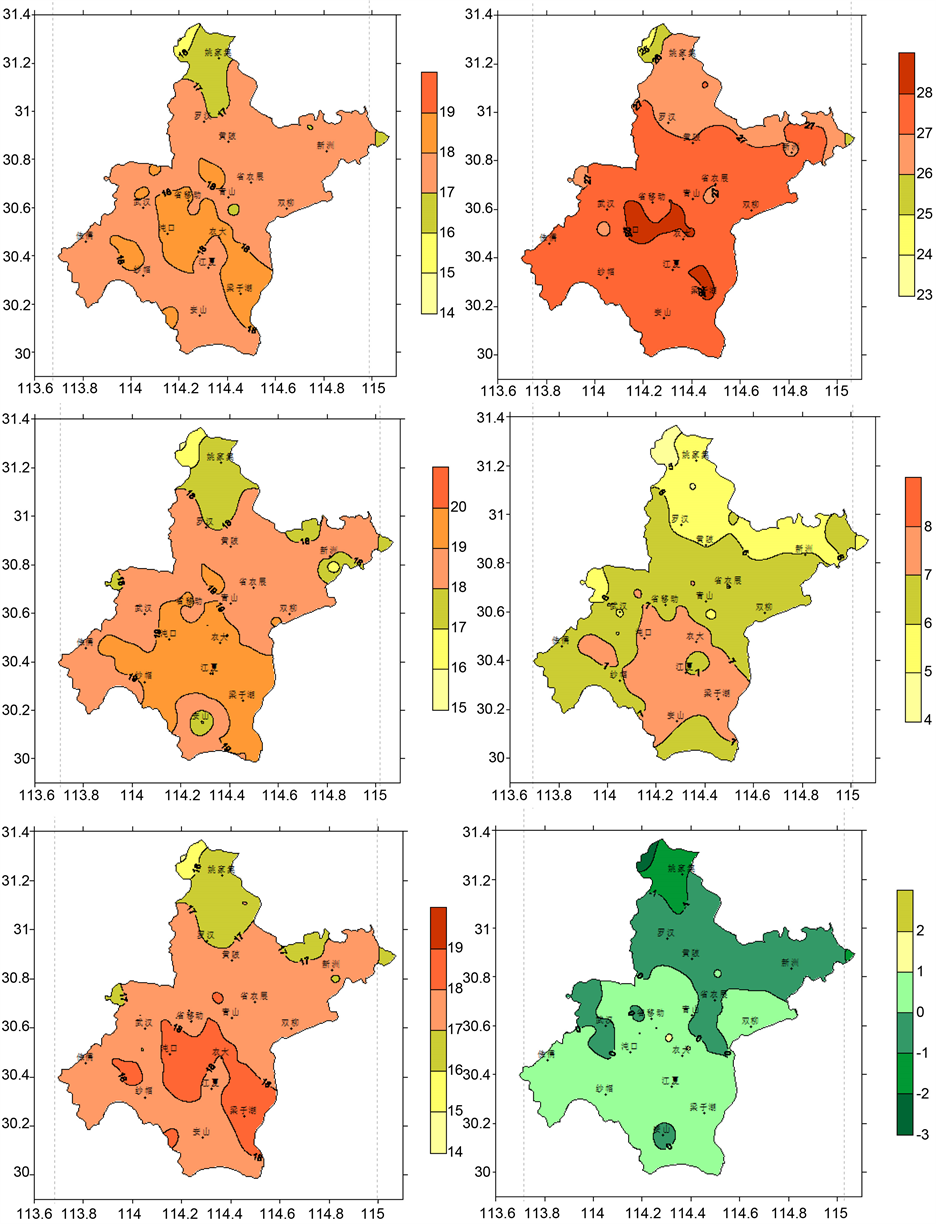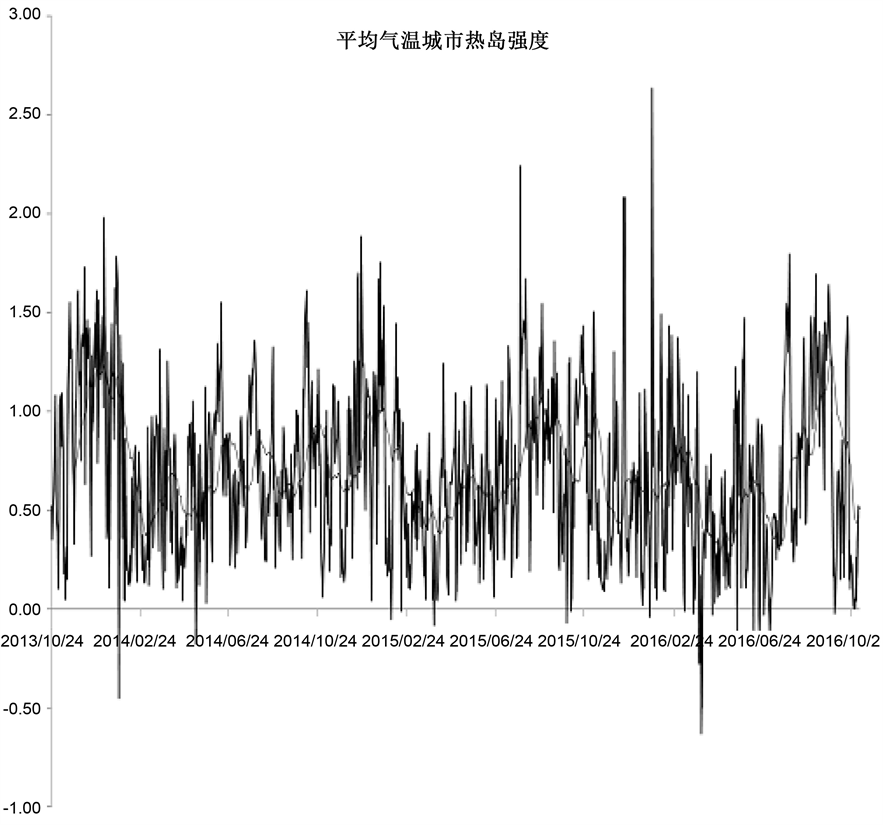﻿ 武汉市城市热岛气象等级标准研究 Study on Meteorological Grade Standard of Urban Heat Island in Wuhan City

Climate Change Research Letters
Vol.07 No.04(2018), Article ID:26084,6 pages
10.12677/CCRL.2018.74028

Study on Meteorological Grade Standard of Urban Heat Island in Wuhan City

Jing Pang1,2, Huosheng Liu2, Kai Yuan2, Jun Qin1, Ming Li2, Yingchun Xu2

1China University of Geosciences (Wuhan), Wuhan Hubei

2Wuhan Meteorological Bureau, Wuhan HubeiReceived: Jul. 4th, 2018; accepted: Jul. 19th, 2018; published: Jul. 26th, 2018ABSTRACT

By analyzing the distribution of air temperature (daily maximum temperature, daily minimum temperature, and daily average temperature) in different seasons and the whole year of automatic weather stations in Wuhan from 2013 to 2016, the temporal and spatial distribution characteristics of air temperature in Wuhan are obtained. Through investigation and research and based on GIS, the representative sites of Wuhan urban area and suburb are selected, the maximum temperature, the lowest temperature and the average temperature are calculated respectively, and the meteorological grade standard of Wuhan urban heat island is developed, which is used to guide people’s life, work and other activities.

Keywords:Urban Heat Island, Represents Site, Heat Island Intensity, Grade

1中国地质大学(武汉)，湖北 武汉

2武汉市气象局，湖北 武汉1. 引言

2. 资料收集与处理

3. 城市热岛特征分析

4. 城市热岛强度及等级划分

${T}_{u}=\frac{\sum _{i=1}^{m}{T}_{i}}{m}$ ，m为城区代表站点的个数Figure 1. Spatial distribution of average air temperature in Wuhan. Upper left: spring, upper right: summer, middle left: autumn, middle right: winter, lower left: annual average, lower right: annual average difference

${T}_{s}=\frac{\sum _{j=1}^{n}{T}_{j}}{n}$ ，n为郊区代表站点的个数

${T}_{u}^{k}={T}_{u}^{k}-{T}_{s}^{k},k=\mathrm{max},\mathrm{min},\text{ave}$

${T}_{UHI}=\text{Max}\left({T}_{u}^{\mathrm{max}},{T}_{u}^{\mathrm{min}},{T}_{u}^{ave}\right)$Figure 2. Time series diagram of urban heat island intensity with average temperatureTable 1. Frequency of urban heat island intensity in various areasTable 2. Meteorological grade standard of urban heat island

5. 小结

Study on Meteorological Grade Standard of Urban Heat Island in Wuhan City[J]. 气候变化研究快报, 2018, 07(04): 252-257. https://doi.org/10.12677/CCRL.2018.74028

1. 1. 李丽光, 王宏博, 贾庆宇, 等. 辽宁省城市热岛强度特征及等级划分[J]. 应用生态学报, 2012, 23(5): 1345-1350.

2. 2. 李晓敏, 曾胜兰. 成都、重庆城市热岛效应特征对比[J]. 气象科技, 2015, 43(5): 888-897.

3. 3. 李丽光, 王宏博, 赵梓淇, 等. 站点选择对城市热岛效应的影响分析[J]. 气象与环境学报, 2013, 29(2): 54-60.